# Multiple DS18B20 Temp sensors interfacing with Raspberry Pi

Interfacing Nine DS18B20 Temperature sensors with Raspberry Pi and Displaying sensor data Using Python

IntermediateFull instructions provided4 hours14,194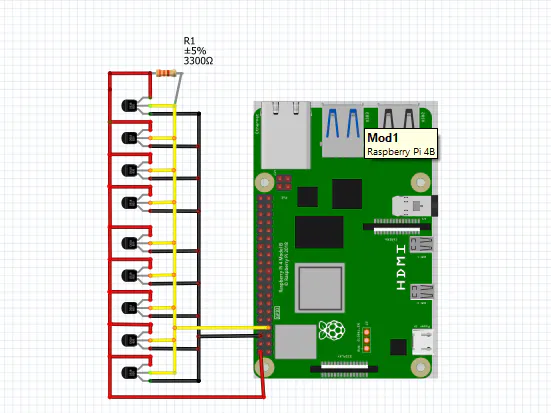## Things used in this project

### Hardware components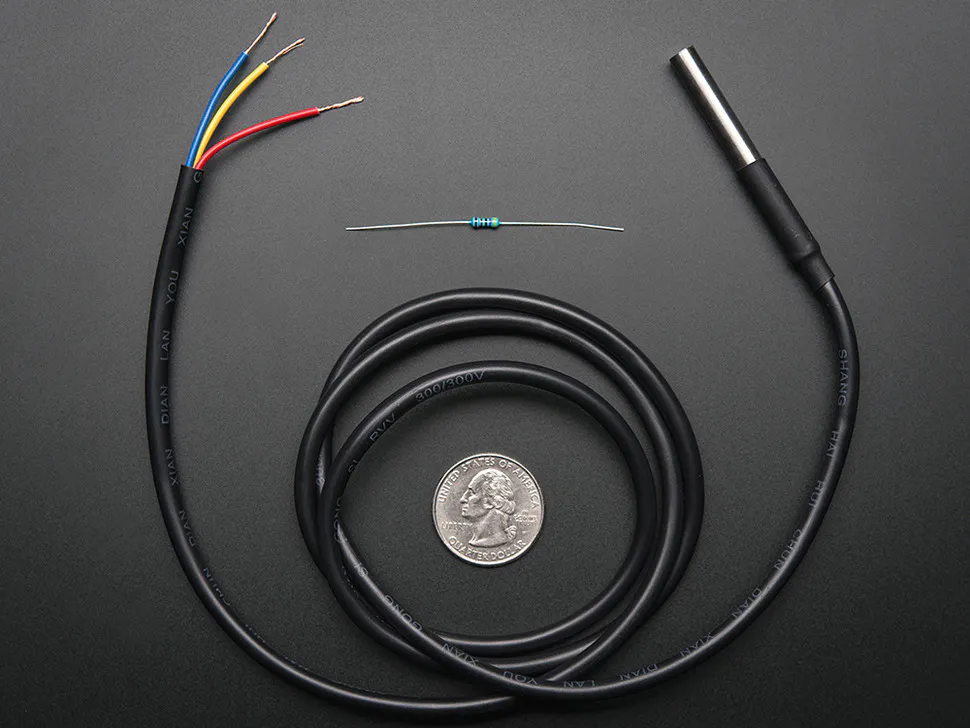Adafruit Waterproof DS18B20 Digital temperature sensor
×9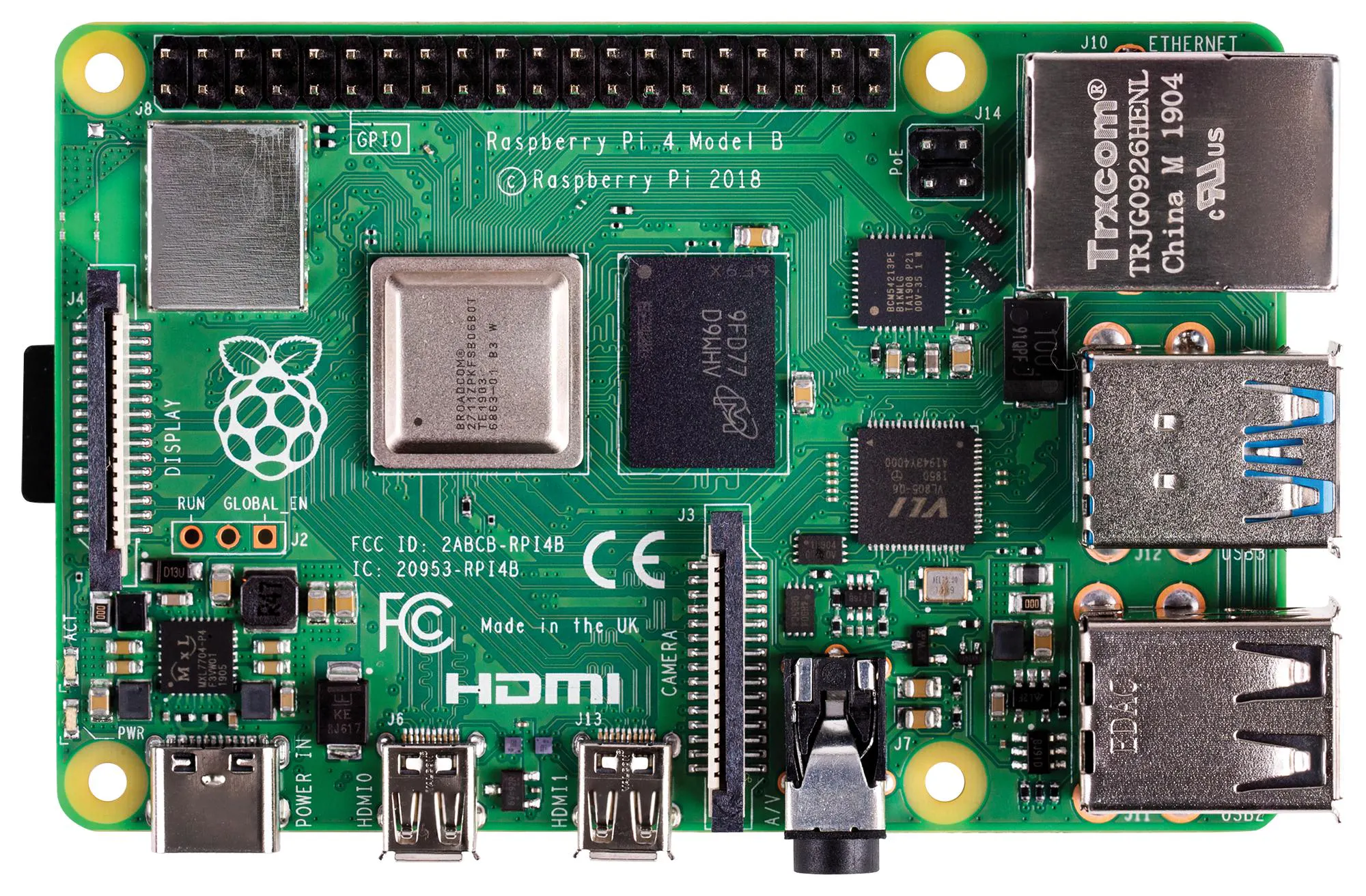Raspberry Pi 4 Model B
×1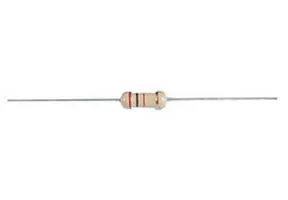Through Hole Resistor, 3.3 kohm
×1

### Software apps and online servicesRaspberry Pi Raspbian

### Hand tools and fabrication machinesSoldering iron (generic)Wire Stripper & Cutter, 18-10 AWG / 0.75-4mm² Capacity Wires

## Schematics

### Schematics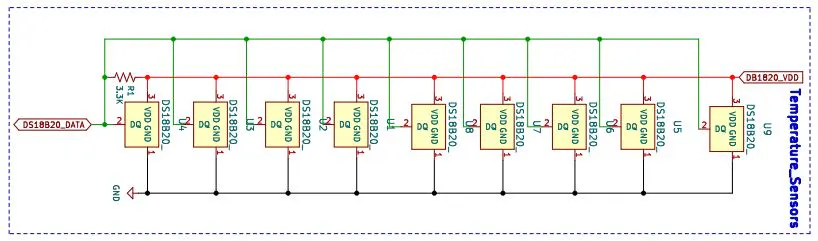### Schematics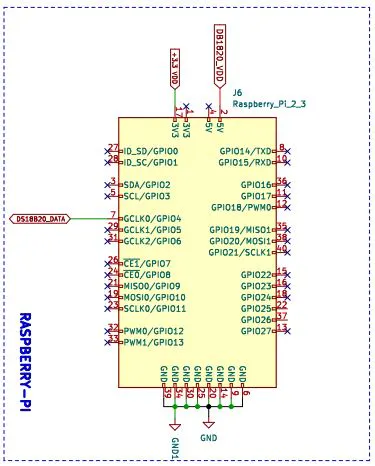### Schematics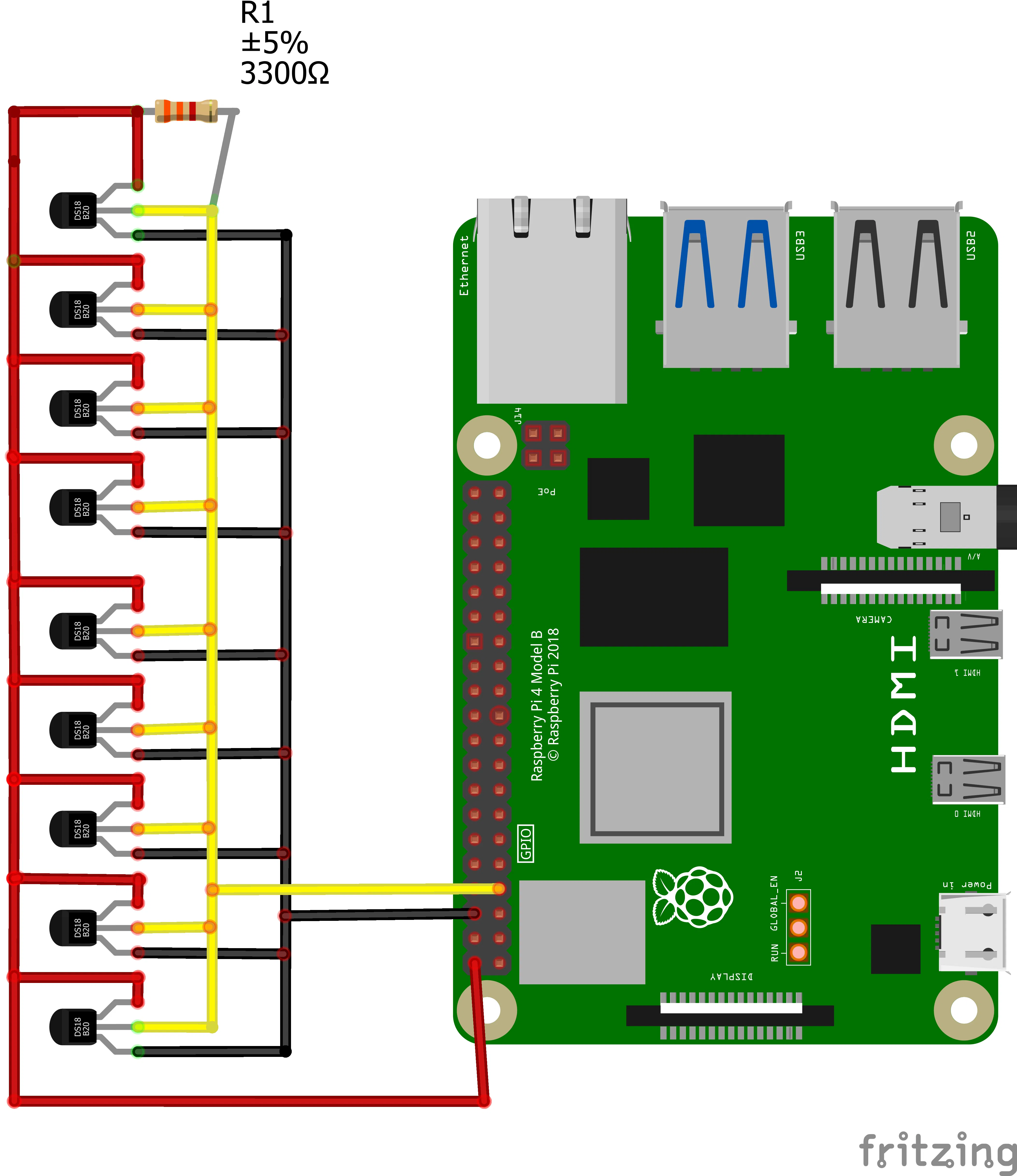## Code

### Project Code

Python
Python 3 is used For Programming
```import os
import glob
import time

# These  lines mount the device:
os.system('modprobe w1-gpio')
os.system('modprobe w1-therm')

base_dir = '/sys/bus/w1/devices/'
# Get all the filenames begin with 28 in the path base_dir.
device_folder = glob.glob(base_dir + '28*')
device_folder1 = glob.glob(base_dir + '28*')
device_folder2 = glob.glob(base_dir + '28*')
device_folder3 = glob.glob(base_dir + '28*')
device_folder4 = glob.glob(base_dir + '28*')
device_folder5 = glob.glob(base_dir + '28*')
device_folder6 = glob.glob(base_dir + '28*')
device_folder7 = glob.glob(base_dir + '28*')
device_folder8 = glob.glob(base_dir + '28*')

device_file = device_folder + '/w1_slave'
device_file1 = device_folder1 + '/w1_slave'
device_file2 = device_folder2 + '/w1_slave'
device_file3 = device_folder3 + '/w1_slave'
device_file4 = device_folder4 + '/w1_slave'
device_file5 = device_folder5 + '/w1_slave'
device_file6 = device_folder6 + '/w1_slave'
device_file7 = device_folder7 + '/w1_slave'
device_file8 = device_folder8 + '/w1_slave'

name_file = device_folder+'/name'
f = open(name_file,'r')
#print('f:',f)

name_file1 = device_folder1+'/name'
g = open(name_file1,'r')
#print('g:',g)

name_file2 = device_folder2+'/name'
h = open(name_file2,'r')
#print('h:',h)

name_file3 = device_folder3+'/name'
i = open(name_file3,'r')
#print('i:',i)

name_file4 = device_folder4+'/name'
j = open(name_file4,'r')
#print('j:',j)

name_file5 = device_folder5+'/name'
k = open(name_file5,'r')
#print('k:',k)

name_file6 = device_folder6+'/name'
l = open(name_file6,'r')
#print('l:',l)

name_file7 = device_folder7+'/name'
m = open(name_file7,'r')
#print('m:',m)

name_file8 = device_folder8+'/name'
n = open(name_file8,'r')
#print('n:',n)

f = open(device_file, 'r')
#print('raw_f',lines)
f.close()
return lines

g = open(device_file1, 'r')
#print('raw_g',lines1)
g.close()
return lines1

h = open(device_file2, 'r')
#print('raw_h',lines2)
h.close()
return lines2

i = open(device_file3, 'r')
#print('raw_i',lines3)
i.close()
return lines3

j = open(device_file4, 'r')
#print('raw_j',lines4)
j.close()
return lines4

k = open(device_file5, 'r')
#print('raw_k',lines5)
k.close()
return lines5

l = open(device_file6, 'r')
#print('raw_l',lines6)
l.close()
return lines6

m = open(device_file7, 'r')
#print('raw_m',lines7)
m.close()
return lines7

n = open(device_file8, 'r')
#print('raw_n',lines8)
n.close()
return lines8

#converting the temperature data to human readable form

while lines.strip()[-3:] != 'YES':
equals_pos = lines.find('t=')
temp_string = lines[equals_pos+2:]
temp_c = float(temp_string) / 1000.0
temp_f = temp_c * 9.0 / 5.0 + 32.0
return temp_c, temp_f

while lines1.strip()[-3:] != 'YES':
equals_pos1 = lines1.find('t=')
temp_string1 = lines1[equals_pos1 +2:]
temp_c1 = float(temp_string1) / 1000.0
temp_f1 = temp_c1 * 9.0 / 5.0 + 32.0
return temp_c1, temp_f1

while lines2.strip()[-3:] != 'YES':
equals_pos2 = lines2.find('t=')
temp_string2 = lines2[equals_pos2 +2:]
temp_c2 = float(temp_string2) / 1000.0
temp_f2 = temp_c2 * 9.0 / 5.0 + 32.0
return temp_c2, temp_f2

while lines3.strip()[-3:] != 'YES':
equals_pos3 = lines3.find('t=')
temp_string3 = lines3[equals_pos3 +2:]
temp_c3 = float(temp_string3) / 1000.0
temp_f3 = temp_c3 * 9.0 / 5.0 + 32.0
return temp_c3, temp_f3

while lines4.strip()[-3:] != 'YES':
equals_pos4 = lines4.find('t=')
temp_string4 = lines4[equals_pos4 +2:]
temp_c4 = float(temp_string4) / 1000.0
temp_f4 = temp_c4 * 9.0 / 5.0 + 32.0
return temp_c4, temp_f4

while lines5.strip()[-3:] != 'YES':
equals_pos5 = lines5.find('t=')
temp_string5 = lines5[equals_pos5 +2:]
temp_c5 = float(temp_string5) / 1000.0
temp_f5 = temp_c5 * 9.0 / 5.0 + 32.0
return temp_c5, temp_f5

while lines6.strip()[-3:] != 'YES':
equals_pos6 = lines6.find('t=')
temp_string6 = lines6[equals_pos6 +2:]
temp_c6 = float(temp_string6) / 1000.0
temp_f6 = temp_c6 * 9.0 / 5.0 + 32.0
return temp_c6, temp_f6

while lines7.strip()[-3:] != 'YES':
equals_pos7 = lines7.find('t=')
temp_string7 = lines7[equals_pos7 +2:]
temp_c7 = float(temp_string7) / 1000.0
temp_f7 = temp_c7 * 9.0 / 5.0 + 32.0
return temp_c7, temp_f7

while lines8.strip()[-3:] != 'YES':
equals_pos8 = lines8.find('t=')
temp_string8 = lines8[equals_pos8 +2:]
temp_c8 = float(temp_string8) / 1000.0
temp_f8 = temp_c8 * 9.0 / 5.0 + 32.0
return temp_c8, temp_f8

while True:
#READING TEMPERATURE DATA AND PRINTINTING THE VALUES OF INDIVIDUAL SENSOR